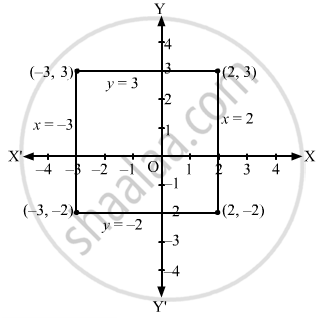Advertisement Remove all ads

# Draw the Lines X = − 3, X = 2, Y = − 2, Y = 3 and Write the Coordinates of the Vertices of the Square So Formed. - Mathematics

Answer in Brief

Draw the lines x = − 3, x = 2, y = − 2, y = 3 and write the coordinates of the vertices of the square so formed.

Advertisement Remove all ads

#### Solution

The lines x = − 3 and x = 2 are parallel to the y-axis. They pass through (−3, 0) and (2, 0), respectively.
Similarly, the lines y = − 2, y = 3 are parallel to the x-axis. They pass through (0, −2) and (0, 3), respectively.
The lines x = − 3, x = 2, y = − 2 and y = 3 are drawn as shown in the following figure.Clearly, the coordinates of the square that is formed are (2, 3), (−3, 3), (−3, −2) and (2, −2).

Concept: Straight Lines - Equation of Family of Lines Passing Through the Point of Intersection of Two Lines
Is there an error in this question or solution?
Advertisement Remove all ads

#### APPEARS IN

RD Sharma Class 11 Mathematics Textbook
Chapter 23 The straight lines
Exercise 23.2 | Q 4 | Page 17
Advertisement Remove all ads
Advertisement Remove all ads
Share
Notifications

View all notifications

Forgot password?